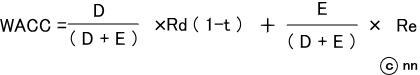# nn的BLOGnn77.exblog.jp

カレンダー
 S M T W T F S 1 2 3 4 5 6 7 8 9 10 11 12 13 14 15 16 17 18 19 20 21 22 23 24 25 26 27 28 29 30

## Weighted-Average Cost of Capital / Capital Asset Pricing Model (BEC: USCPA)

Saturday February 4, 2006

【14:00】

If you are a CFO of a company, you have to establish a financial policy to design the ideal Balance Sheet of your company. But whatever policy you may establish, you have to consider the cost it takes to establish your policy.

Weighted-Average Cost of Capital: WACC

A company has two methods for financing: Debt & Equity.
From the view point of a company, Debt must be repaid with interests by due. But that’s all. Equity, on the other hand, doesn’t have to be repaid to anybody; instead many rights are provided to shareholders: right to dividends, right to distribution of assets on dissolution, etc.
Both Debt and Equity costs some expenses for a company, you, as CFO, have to figure out figure out the most ideal proportion of Debt & Equity in your balance sheet.
WACC is, as it were, the total cost of Debt \$ Equity. So your policy must decrease WACC as much as possible.
The formula of WACC isD: Amount of Debt
E: Amount of Equity
t: Tax Rate
Rd: Cost of Debt (interest, etc)
Re: Cost of Equity (expected dividends payout, etc)

The above formula is simple! It just prorates Cost of Debt and Cost of Equity based on the amount of Debt and Equity. “t” is incorporated as an interest-expense-deducting f factor, because

1) interest expense is not merely cost, but it also contributes the reduction of tax expense because interest expense decreases Taxable Net Income.

2) Thus the above effect was taken into consideration in the above formula. In other word, the cost of Debt is lower than face interest rate because int.expense has a favorable characteristics at the same time, which is to reduce Tax Expense.

By the way, Rd, Cost of Debt, is easy to imagine. It consists of interest expense, and the fees for guarantors, and so forth. But can you imagine how to determine Re, Cost of Equity?

There’re several models to calculate the cost of equity. I introduce one of them below. It is Capital Asset Pricing Model.

Capital Asset Pricing Model: CAPM

You, as CFO, have to consider the Cost of Equity to figure out WACC. The Cost of Capital is, if we see it from the investors’ viewpoint, the Required (Expected) Rate of Return for the investment. Investors take some risk for their investment so they require some return for their risk.

Following is the link to a graph that illustrates such a required rate of return.

http://teachmefinance.com/securitymarketline.html

Risk Free Rate (of Return) indicates the return that investors can get without running any risk (like time-deposit in a bank.) In order for an investor to run a risk to purchase Stock X, for example, Stock X must be more attractive than Risk-Free Rate financial instruments like deposit in a bank. (By the way, this attractiveness is the Cost of Equity from the viewpoint of the issuer company.)
As is indicated the graph above, this attractiveness, (i.e. Required Rate of Return) is computed by multiplying Market Risk Premium by beta coefficient (β).

#### R = Rf + β×( Rm －Rf )

Rf: Risk Free Rate of Return

Beta coefficient：β is an index that indicates how sensitive Stock X is to the whole market, or how strong the relation between Stock X and the whole market is.

( Rm －Rf )＝Market Risk Premium:
= Required Rate (of Return) from that MARKET ― Risk Free Rate
If I rephrase in my own way, Market Risk Premium is the compensation required by the investors to jump into the relatively risky market. And, since βis such an indicator, the higher the βof the Stock is, the higher the compensation (required or expected return from the investment) must be.

This is the way how an investor can determine the Required Rate of Return from the Equity investment. And this method can also be used by a CFO of a company to assess the Cost of Equity (finance) , for example, to figure out WACC.

タグ：
by | 2006-02-04 13:49 | ＞BEC